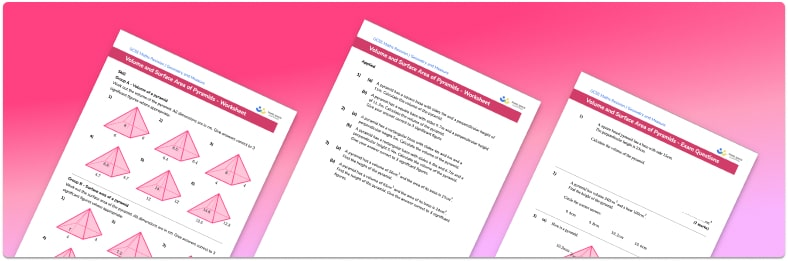# Volume And Surface Area Of A Pyramid WorksheetHelp your students prepare for their Maths GCSE with this free volume and surface area of pyramids worksheet of 26 questions and answers

• Section 1 of the volume and surface area of pyramids worksheet contains 18 skills-based volume and surface area of pyramids questions, in 3 groups to support differentiation
• Section 2 contains 4 applied volume and surface area of pyramids questions with a mix of worded problems and deeper problem solving questions
• Section 3 contains 4 foundation and higher level GCSE exam style volume and surface area of pyramids questions
• Answers and a mark scheme for all volume and surface area of pyramids questions are provided
• Questions follow variation theory with plenty of opportunities for students to work independently at their own level
• All questions created by fully qualified expert secondary maths teachers
• Suitable for GCSE maths revision for AQA, OCR and Edexcel exam boards

• This field is for validation purposes and should be left unchanged.

You can unsubscribe at any time (each email we send will contain an easy way to unsubscribe). To find out more about how we use your data, see our privacy policy.

### Volume and surface area of pyramids at a glance

A pyramid is a 3d shape. The base of a pyramid is a polygon; every other face of a pyramid is a triangle which meet at a point at the top, usually referred to as the apex. Some common types of pyramids are triangular pyramids, square pyramids (or square base pyramids), rectangular pyramids, pentagonal pyramids, and hexagonal pyramids.

If the apex lies directly above the centre of the base, the pyramid is called a right pyramid. If the apex does not lie directly above the centre of the pyramid, it is called an oblique pyramid.

The volume of a pyramid is calculated using the formula: V= 1 over 3, multiplied by the area of the base, multiplied by the perpendicular height of the pyramid.

The total surface area of a pyramid (also called lateral surface area) is found by adding the area of the base and the area of each triangular face together. The area of each triangular face can be found by multiplying the length of their base by the perpendicular height of the triangular face, and dividing by 2. The triangular faces may not have the same area as they may not have the same dimensions.

You will need to use Pythagoras’ Theorem to calculate the perpendicular height of a face if you are given the slant height between the apex and another vertex.

Looking forward, students can then progress to additional geometry worksheets, for example the volume and surface area of prisms worksheet, the volume and surface area of cones worksheet, or the volume and surface area of spheres worksheet.For more teaching and learning support on Geometry our GCSE maths lessons provide step by step support for all GCSE maths concepts.

## Do you have KS4 students who need more focused attention to succeed at GCSE?There will be students in your class who require individual attention to help them succeed in their maths GCSEs. In a class of 30, it’s not always easy to provide.

Help your students feel confident with exam-style questions and the strategies they’ll need to answer them correctly with our dedicated GCSE maths revision programme.

Lessons are selected to provide support where each student needs it most, and specially-trained GCSE maths tutors adapt the pitch and pace of each lesson. This ensures a personalised revision programme that raises grades and boosts confidence.

Find out more